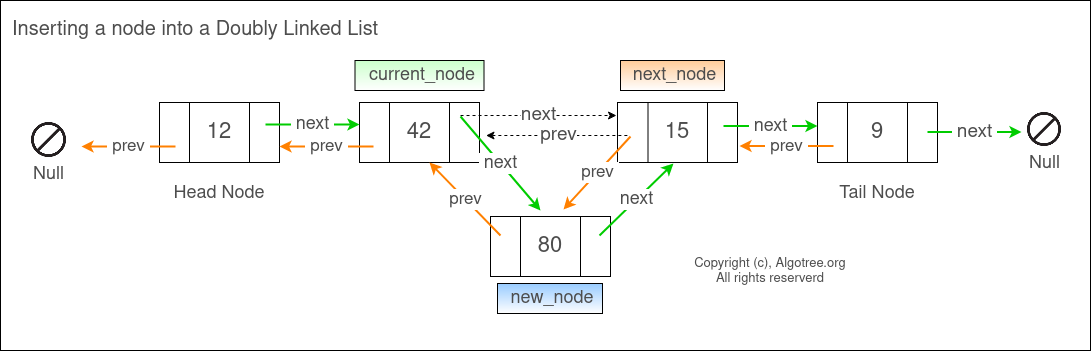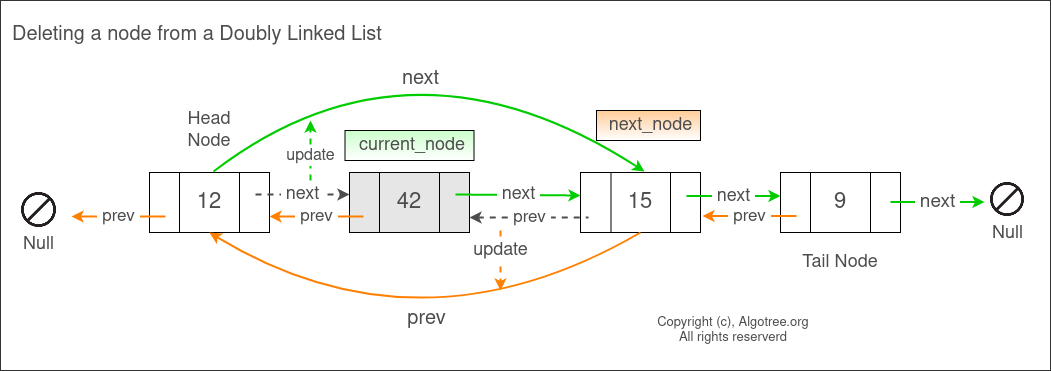Doubly Linked List : Insert, Append and Delete

- Doubly linked list consists of dynamically allocated nodes that are linked to each other.
- Every node in a doubly linked list has some data and 2 node pointers ( previous and next ).
- Previous pointer of a node points to the previous node.
- Next pointer of a node points to the next node.
- The nodes in a doubly linked list are not contiguous in memory but are located randomly in memory locations allocated at runtime.
- Since the nodes in a linked list are allocated memory at runtime, a linked list can grow or shink depending on the need.Insert Operation
To insert into a doubly-linked list, we need to know the node after which we need to insert a new_node.
1. Traverse the linked list and store the location of the node ( into current_node ) after which a new_node is to be inserted.
2. If the current_node is not Null then
Create a new_node that is to be inserted.
Store the location of the node that is next to the current_node i.e ( next_node = current . next )
Update the next link of the current_node by pointing it to the new_node.
Point new_node’s prev link to the current_node and new_node’s next link to next_node.
If next_node is not Null then
Update the prev link of the next_node to point it to the new_node.
Example : In the below doubly-linked list, a new node with data 80 is inserted after node (42).Append Operation
To append to a doubly-linked list,
1. Traverse the linked list till the end. Store the location of the last node into current_node.
Create a new_node to be appended.
Update the next link of the current_node by pointing it to the new_node.
Point new_node’s prev link to the current_node.

Delete Operation
(This algorithm deletes the first node with the matching data.)
1. If the node to be deleted matches with the head node i.e head.data = data to be deleted, then
Store the location of the node to the right of head into next_node.
Update the left link of next_node by pointing it to NULL.
2. Else travere the list from the head node till the node to be deleted (current_node) is found.
If current_node is not NULL then
Update the next link of the node that is previous to the current_node to point at current_node’s next node.
If the node next to the current_node is not NULL then
Update the prev link of the node next to the current_node to point at current_node’s previous node.
Delete the current_node.
Example : In the below doubly-linked list node with data 42 is deleted.C++ Program to demonstrate create, insert, append and delete operations on a doubly linked list.

#include<iostream>

using namespace std;

class Node {

public:
int data;
Node* next;
Node* prev;
Node (int arg_data) : data (arg_data), next (nullptr), prev (nullptr)
{}
};

private:
public:

}

}

}

// Insert a new node into the doubly-linked list after the first found node.
void Insert (int data, int after) {

// Traverse the linked list till the node is found after
// which the data is to be insert
while (current != nullptr && current->data != after) {
current = current->next;
}

if (current != nullptr) {
Node * temp = new Node(data);
// Save the location of node after current in next_node.
Node * next_node = current->next;
// Point current's link to the new node to be inserted.
current->next = temp;

// Point new node's next link (to the next node location previously stored).
// left link to the current node.
temp->prev  = current;
temp->next = next_node;

// Point next node's prev to the newly added node i.e temp.
if (next_node != nullptr)
next_node->prev = temp;
}
}

// Delete the first node matching the data from the doubly linked list.
void Delete (int data_to_be_deleted ) {

// If the head node is to be deleted, then the node to the next of head becomes the head node.
if (head->data == data_to_be_deleted ) {
} else {
while (current->data != data_to_be_deleted ) {
current = current->next;
}
// current is to be deleted.
if (current != nullptr) {
current->prev->next = current->next; // Update the next link of the node previous of current
if (current->next != nullptr) {
current->next->prev = current->prev; // Update the previous link of the node to the next of current
}
delete current;
}
}
}

// Append a node to the doubly linked list.
void Append (int data) {

while (current->next != nullptr) {
current = current->next;
}
Node* temp = new Node(data);
current->next = temp;
temp->prev = current;
}

// Display all the nodes in the doubly linked list.
void Display () {

cout << "[ ";
while (current != nullptr) {
cout << current->data << " ";
current = current->next;
}
cout << "]";
}

void FreeList () {

}
}
};

int main() {

int data, after, opt = 0;

while (opt != 5) {
cout << "\n\nOptions" << endl;
cout << "0. Create head." << endl;
cout << "1. Insert node." << endl;
cout << "2. Append node." << endl;
cout << "3. Delete node." << endl;
cout << "4. Free the linked list." << endl;
cout << "5. Exit." << endl;
cout << "Enter Option : ";
cin >> opt;
switch (opt) {

case 0:
s.Display();
cout << "\nHead exist." << endl;
} else {
cout << "\nEnter head node (data) : ";
cin >> data;
s.Display();
}
break;

case 1:
s.Display();
cout << "\nEnter new node (data) : ";
cin >> data;
cout << "After node : ";
cin >> after;
s.Insert(data, after);
s.Display();
break;

case 2:
s.Display();
cout << "\nEnter new node (data) : ";
cin >> data;
s.Append(data);
s.Display();
break;

case 3:
s.Display();
cout << "\nEnter node (data) to be deleted : ";
cin >> data;
s.Delete(data);
s.Display();
break;

case 4:
cout << "Freeing the linked list." << endl;
s.FreeList();
break;
}
}
return 0;
}

Output

Options
1. Insert node.
2. Append node.
3. Delete node.
5. Exit.
Enter Option : 0
Enter head node (data) : 10
[ 10 ]

Options
1. Insert node.
2. Append node.
3. Delete node.
5. Exit.
Enter Option : 2
Enter new node (data) : 42
[ 10 42 ]

Options
1. Insert node.
2. Append node.
3. Delete node.
5. Exit.
Enter Option : 1
Linked list [ 10 42 ]
Enter new node (data) : 50
After node : 10
[ 10 50 42 ]

Options
1. Insert node.
2. Append node.
3. Delete node.
5. Exit.
Enter Option : 1
Linked list [ 10 50 42 ]
Enter new node (data) : 80
After node : 42
[ 10 50 42 80 ]

Options
1. Insert node.
2. Append node.
3. Delete node.
5. Exit.
Enter Option : 3
Linked list [ 10 50 42 80 ]
Enter node (data) to be deleted : 10
[ 50 42 80 ]

Options
1. Insert node.
2. Append node.
3. Delete node.
5. Exit.
Enter Option : 3
Linked list [ 50 42 80 ]
Enter node (data) to be deleted : 42
[ 50 80 ]

Options
1. Insert node.
2. Append node.
3. Delete node.
5. Exit.
Enter Option : 2
Linked list [ 50 80 ]
Enter new node (data) : 100
[ 50 80 100 ]

Options
1. Insert node.
2. Append node.
3. Delete node.
5. Exit.
Enter Option : 4

Options